An Economic Analysis of Alternative Damage Rules for Breach of Contract*

by

David D. Friedman

John M. Olin Fellow

University of Chicago Law School

The party who breaches a contract may be required to make the victim as well off as if the contract had been fulfilled (expectation) or never signed (reliance). It has been argued that expectation is economically superior because it generates the correct incentive to breach. But damage rules also affect the incentive to sign. When P=MC both rules imply the same outcome. If P!= MC because of imperfect competition the analysis reduces to a special case of third order price discrimination; which rule is superior is indeterminate. Where P!= MC because of uncertainty, expectation is superior if information is symmetric.

March 31, 1989

Notes to Web Version

1. If I were writing the article today, I would take more care to point out that the distinction between expectation and reliance damages is closely related to the problems of moral hazard and adverse selection in insurance. We solve the problem of moral hazard by making the actor bear the full costs of his acts--as expectation damages makes the breaching party bear the full costs of his breach. We solve the problem of adverse selection by making the party with the private information fully insure the other party against the risk the information relates to. That way efficient and only efficient transactions take place, since the knowledgable party knows the risks and bears them and the ignorant but insured party does not care what the risks are. Similarly, under reliance damages, the party with superior knowledge as to the proability that he will wish to breach is insuring the other party--making sure that he is no worse off signing and suffering breach than not signing. Just as in the insurance case, we have no guarantee that the same rule will optimize on both margins.

2. This article is being posted on the Web with the assent of the Journal of Law and Economics, for which I am grateful. I believe it is very close to identical to the published version.

An Economic Analysis of Alternative Damage Rules for Breach of Contract

I: Introduction

I order six gross of customized battens from you, paying in advance. Before the delivery date I call you up and cancel the order. How much of the price should you be obliged to give back to me, and how much are you entitled to keep as compensation for my refusal to fulfill my half of the purchase contract? More generally, what is the appropriate rule for determining the damages for breach of contract?

In discussions of this question, both in the traditional legal and the more recent economic literature, one important issue is whether a buyer who breaches should be required to reimburse the seller for his lost profit. Put differently, the question is whether the damages should be sufficient to make the seller as well off as he would have been if the buyer had failed to breach ("expectation damages), or only as well off as he would have been if the buyer had not purchased in the first place ("reliance damages").

Under the former rule, the seller would refund to the buyer whatever money was saved by not completing the battens, including anything he could get for them in their uncompleted condition. He would then be as well off as if the buyer had not breached the contract--he would receive his expectation. Under the latter rule, the seller would figure out how much he had spent so far on producing the battens net of what he could sell them for. That sum, called his reliance, would be subtracted from what he had been paid, and the remainder returned to the buyer. The seller, having been compensated for the cost of having the contract made and broken, would be as well off as if the battens had never been ordered.

Much of the literature on damage rules for breach of contract argues that an expectation rule leads to an economically more efficient result than does a reliance rule and that damages should therefore include compensation for the seller's lost profits. This article argues that that conclusion is mistaken. Where the two rules lead to different results, there is no general reason to prefer the rule that reimburses the seller for his lost profits. In some cases, that rule produces a less efficient outcome than the alternative. In general, which rule is more efficient depends on the details of the particular situation.

In order to focus on the lost profits issue, I am deliberately avoiding a number of the complications discussed in the literature, such as how to measure the amount of damage and how to prevent the damage rule from generating inefficient levels of reliance. In all of the examples I will consider, the amount of reliance is fixed; it cannot be varied by either party. I assume that buyers and sellers are risk neutral, that the market interest rate is zero, that the court can costlessly measure the producer's costs, and that resale by the buyer is prohibitively costly. I limit my discussion to contracts to purchase goods or services, and further limit it to cases in which reliance is by the seller and the decision to breach is made by the buyer. The applicability of the conclusions to a much wider range of situations will, I think, be clear.

As has been pointed out by a number of writers, the issue of lost profits only arises when there are profits to lose. Under conditions of perfect competition, price normally equals marginal cost; the producer is indifferent between selling and not selling an additional unit. In this case, the damage rule "make the seller as well off as if the purchaser had not breached" leads to the same result as the rule "make the seller as well off as if the purchaser had not bought."

The issue of lost profits arises when for some reason price is not equal to marginal cost. One such situation, imperfect competition, is analyzed in part III of this article, and another, perfect competition with uncertain cost, in part IV. Part V summarizes the conclusions.

II: The Case for Lost Profits and What is Wrong With It

The argument in favor of an expectation rule can be stated very simply. An individual will breach a contract if his private benefits from breach are greater than his private costs. He ought to breach (from the standpoint of economic efficiency) if social benefits--total benefits to everyone affected, himself included--are greater than social costs. The seller's lost profit is one of the costs of breach. By requiring the purchaser to bear this cost in the form of damages, we add it to the private costs which go into his decision. If his benefit from breach is still greater than his cost, then he ought to breach--doing so produces a net gain--and he will. If not, he ought not to, and will not. So including the seller's lost profits in the damages leads to efficient breach.

While this rule leads to efficient breach, it does not follow that it is an efficient rule. The damage rule sets the cost of breaching the contract and thus the buyer's incentive to avoid doing so. There are, however, two ways of avoiding breach. One is not to breach the contract and the other is not to sign it in the first place. The expectation rule provides the right incentive on only one of the two margins.

In some circumstances, as we shall see in Parts III and IV, the purchase price provides the correct incentive on the other margin, making the argument for expectation damages a valid one. In other circumstances it does not. In the latter case, there are advantages to both the reliance and the expectation rules; which is preferable depends on the details of the situation.

III: Imperfect Competition

In this part of the article, we analyze the effect of different damage rules under circumstances of imperfect competition, concentrating on the simple case of a single price monopoly. In order to do so, we must consider more than the direct effect of the rule on the decision to breach or not to breach. If a purchaser knows that circumstances may arise in which he will want to breach the contract, then a change in the damage rule, like a change in price, affects whether and how much he buys, shifting his demand curve. A change in the damage rule also changes the seller's profit function by affecting how much he gets in case of breach. The result is to alter the monopolist's profit-maximizing price, the quantity initially ordered, and the quantity ultimately consumed. All of these effects must be taken into account in deciding which damage rule to prefer.

In the case of imperfect competition, an expectation damages rule fixes only part of the problem. The purpose of the rule is to give the consumer the right incentive to breach or not to breach. But the consumer still has the wrong incentive to buy or not to buy. The efficient rule for that decision is to buy if the value of a unit of the good to the consumer is greater than the cost of producing it. The rule the consumer will follow, however, is to buy if the value is greater than the price. If the price of the good is greater than the cost of producing it, the consumer will sometimes fail to buy even though, from the standpoint of economic efficiency, he should--whenever the value of the good to him is more than its marginal cost but less than its price. This inefficiency is the traditional economic argument against monopoly. If the price were not greater than the cost of production, expectation and reliance rules would yield the same result, so the inefficiency of monopoly pricing is intimately related to the evaluation of the two rules.

The same fact--price greater than cost--that makes the consumer buy too little also, under reliance damages, makes him breach too often. The consumer fails to buy if, at the time of purchase, the value to him of the good is less than its price. He fails to take delivery if, between the time of purchase and the time of breach, something happens that lowers the good's value to him below its price. In both cases, the efficient decision would be to consume the good if its value is greater than the marginal cost of producing it.

The argument that implies that efficient breach requires the consumer to pay damages equal to lost profits if he breaches also implies that he should pay the same damages if he fails to purchase in the first place! That decision also results in lost profit to the seller, and will be made efficiently only if that cost is taken into account by the buyer.

Single Price Monopoly

The argument can be made more concrete by applying it to a specific case. Assume a producer with a monopoly of a particular good. His marginal cost curve is horizontal; for simplicity fixed cost is assumed to be zero. He sells at a single price to a large number of identical consumers. Units are customized; a unit produced for one consumer cannot be resold to another.

The good is produced in a two step process. The first stage, which occurs after the order but before the consumer decides whether to breach, costs the producer R per unit; this is his reliance. If the contract is breached, the unit is junked; it has no scrap value. Completing the good costs an additional MC-R per unit.

Each consumer faces an uncertain future. There is a probability p that, between the time he orders and the time he takes delivery, an event will occur that will lower the value to him of the good. The event occurs separately for each consumer and the probabilities are independent; since there are many consumers, the fraction for whom the event does occur will be very close to p. In Figures 1 to 7, p is 1/2.

Each consumer's total value function for the good is TV1(Q) if the event does not occur and TV2(Q) if it does; the corresponding marginal value functions are MV1 and MV2. Since the event lowers the value of the good, we assume that MV2 is below MV1 for all values of Q. MV1,2 are assumed continuous and monotonically decreasing.

We consider two possible damage rules: reliance and expectation. Under the reliance rule, a consumer who takes delivery of fewer units than he ordered must reimburse the seller only for his reliance; the seller refunds to the buyer P-R for each unit which the buyer has decided he does not want. Under the expectation rule, the consumer receives back only MC-R for each unit he chooses not to accept. In this case, the penalty for breach is the difference between the contract price and the cost of completing the good--the breaching consumer must reimburse the seller for his lost profits.

I will show that the problem defined by TV1, TV2, and R can be be converted, under either rule, into an equivalent problem with new total value curves TV*1, TV*2 and zero reliance. By solving that problem in the case where MV*1 and MV*2 are parallel straight lines, a situation that happens to lead to a particularly simple solution, I will show, for each rule, that there exist circumstances in which that rule produces the superior outcome.

Reliance Damages

We start with reliance damages. The consumer orders a quantity Q1. If the event does not occur, he accepts delivery and receives a benefit TV1(Q1) at a cost PQ1. If the event does occur, he breaches a quantity equal to (Q1-Q2), consumes Q2, and receives a benefit TV2(Q2) at a cost PQ2 + R(Q1-Q2); the final term is the damage payment. If NBr is the consumer's net benefit under a reliance rule, we have:

NBr(Q1,Q2)= (1-p)[TV1(Q1)-PQ1]+p[TV2(Q2) - PQ2]-pR(Q1-Q2) (Equation 1)

The consumer chooses Q1, Q2 to maximize NBr. He does so subject to the constraint Q1>= Q2, since he does not have the option of ordering Q1 at a price P and then breaching a negative quantity by ordering additional units at a cost of P-R.

We consider two alternatives: case 1, where the constraint is not binding, and case 2, where it is. We start with case 1, giving us:

0=dNBr/dQ1 = (1-p)[MV1(Q1)-P]-pR (Equation 2)

\ P= MV1(Q1) - Rp/(1-p) = MV*1(Q1) (Equation 3)

0=dNBr/dQ2 =p[MV2(Q2)-P]+pR (Equation 4)

\ P= MV2(Q2) + R = MV*2(Q1) (Equation 5)

Ordinarily an individual's demand curve is equal to his marginal value curve, since he maximizes his net benefit by buying the quantity for which price equals marginal value. We have a similar result here, except that the demand curve showing the quantity consumed in state 1 (event does not occur, probability 1-p) is equal to MV*1(Q1), shifted down from MV1(Q1) by pR/(1-p), while the demand curve for state 2 is the corresponding marginal value curve shifted up by R.

Under the reliance rule, damages for breach just compensate the producer for the cost of producing units that are not accepted; the producer is in the same position as if the consumer had ordered the number of units that he ends up accepting. So we have:

[[pi]] =(1-p)Q1(P-MC) + pQ2(P-MC)=D*(P)(P-MC)

where:

D*(P)= [(1-p)Q1 + pQ2].

The producer makes the same profit as if he had sold a quantity D*(P) at a price P. D*(P) is simply the horizontal weighted average of the demand curves corresponding to MV*1 and MV*2, with weights (1-p), p.

So far we have considered the case in which the constraint Q1>= Q2 is not binding. The consumer orders Q1; if the event occurs he cancels part of the order and gets back P-R on each unit cancelled. If, however, MV2(Q1)>P-R, the consumer will choose not to breach and Equations 2-5 no longer hold. We have instead:

NBr(Q1,Q2)= (1-p)TV1(Q1)+pTV2(Q1) - PQ1

Q2=Q1

Maximizing NBr with respect to Q1 yields:

0=dNBr/dQ1 =[ (1-p)MV1(Q1)+pMV2(Q1)]-P (Equation 6)

\ P= (1-p)MV1(Q1)+pMV2(Q1)=(1-p)MV*1(Q1)+pMV*2(Q1)

Here the consumer, knowing that he is not going to breach, chooses Q1 in the knowledge that he has a probability 1-p of getting TV1(Q1) and a probability p of getting TV2(Q1). His (expected) marginal value curve, and therefore his demand curve D*, is the vertical average of MV1 and MV2 with weights 1-p, p.

The seller knows that, whether or not the event occurs, he will sell the quantity calculated from that demand curve, so he picks his price to maximize his profit on D* in the usual way. The situation is shown on Figure 1, for p=1/2. For quantities for which MV*1 (Q) > MV*2(Q) the constraint Q1>= Q2 is not binding and D* is the horizontal weighted average of MV*1 and MV*2; elsewhere the constraint is binding and D* is the vertical weighted average. At the boundary of the two regions MV*1= MV*2; vertical and horizontal averages are the same, so D* is continuous at the boundary. In order to maximize his profit, the producer calculates marginal revenue from D*, intersects it with MC to find the profit-maximizing (average) quantity, and goes up to D* to find the corresponding price.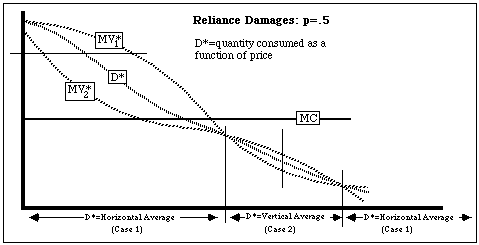Figure 1: Calculating the demand curve faced by a monopoly under a rule of reliance damages.

We have now converted our original problem, with MV1,2 and R>0, into a new problem with MV*1,2 and R=0; we have eliminated R by a shift of the marginal value curves. The new problem yields the same demand curve as the original problem, so the profit-maximizing price, quantity consumed, and profit are the same. From the standpoint of the producer the outcome is identical to the outcome of the original problem.

We may rewrite equation 1 as:

NBr(Q1,Q2) = (1-p)[TV1(Q1)-Rp/(1-p)Q1-PQ1]+p[TV2(Q2)+RQ2 - PQ2]

= (1-p)[TV*1(Q1)-PQ1]+p[TV*2(Q2)- PQ2] (Equation 1')

Here TV*1,2 are the total value curves corresponding to the marginal value curves MV*1,2 . The right hand side of Equation 1' is what total benefit would be if reliance were zero and the marginal value curves were MV*1,2. So the problem defined by MV*1,2 and R=0 is equivalent to the original problem from the standpoint of the consumer as well.

Expectation Damages

The analysis for expectation damages is similar.The essential difference is that, since the buyer must reimburse the seller for his lost profit, he receives back only MC-R for each unit cancelled. So his net benefit is:

NBe(Q1,Q2)= (1-p)[TV1(Q1)-PQ1]+p[TV2(Q2)-PQ2]-p[P-(MC-R)](Q1-Q2) (Equation 7)

As before, we start by considering the case where, if the event happens, the consumer chooses to cancel at least part of his order. Maximizing with respect to Q1 and Q2 we have:

0=dNBe/dQ1 = (1-p)[MV1(Q1)-P]-p[P-(MC-R)] (Equation 8)

\ P= (1-p)MV1(Q1) + p(MC-R)=(1-p)MV*1(Q1) + pMC (Equation 9)

0=dNBe/dQ2 =p[MV2(Q2)-P]+p[P-(MC-R)] (Equation 10)

\ MC= MV2(Q2)+R = MV*2(Q2) (Equation 11)

Looking at Equation 10, we observe that the optimal value of Q2 does not depend on P. If the event happens, the consumer cancels Q1-Q2 units, getting back MC-R on each. He maximizes his net benefit by cancelling back to the point where the marginal value of the last unit equals what he gets for cancelling it.

It is easy to see when the constraint Q1>= Q2 is or is not binding. If the consumer purchases a quantity Q1 such that MV2(Q1)<= MC-R then the constraint is not binding (case 1), otherwise it is (case 2). In the latter case, the consumer orders Q1 and consumes it all, whether or not the event occurs.

Under the reliance rule, the producer's profit depended on how much was consumed. Under the expectation rule, the consumer who chooses to cancel part of his order must make the producer as well off as if he had not cancelled, so the producer's profit depends only on Q1, the quantity ordered. From equation 9, we know that in case 1 that quantity is ordered for which P=(1-p)MV*1(Q1) + pMC. So the producer maximizes his profit by acting as if he were facing a demand curve D*=(1-p)MV*1(Q1) + pMC.

In case 2, everything ordered is consumed. The result is the same as in case 2 under reliance damages. D* is the vertical average of MV1 and MV2 with weights 1-p, p. That is the same as the vertical average of MV*1 and MV*2 with the same weights.

I have now done the same thing under expectation damages that I earlier did under reliance damages--converted a problem with MV1,2 and R>0 into a new problem with MV*1,2 and R=0 and shown that the two problems are equivalent from the standpoint of the producer. Just as in the earlier case, the final step is to show that the problems are also equivalent from the standpoint of the consumer. We rewrite Equation 7 as:

NBe(Q1,Q2) =

=(1-p){ TV1(Q1)-RpQ1/(1-p)-Q1[P+(P-MC)p/(1-p)} +p[TV2(Q2)+RQ2+(P-MC)Q2-PQ2]

=(1-p)[TV*1(Q1)+p[TV*2(Q2)]-PQ1+p(MC)(Q1-Q2) Equation 7'

This is the same net benefit we would get from Equation 7 for R=0, TV1,2=TV*1,2. So the new problem is equivalent to the old from the standpoint of the consumer as well.

Comparing the Two Rules

Figures 1 and 2 show the results from the standpoint of the producer; in each case, he calculates price, quantity, and profit as if he were faced with a demand curve D*. To calculate the effect of each damage rule on price and quantity, we must learn in each case where the marginal revenue curve derived from D* intersects MC. Doing so is complicated by the fact that the two rules imply both different ranges of Q1 for which the constraint Q1>= Q2 is binding and, if it is not binding, different relations between D* and the marginal revenue curves MV1,2 .

Fortunately, the objective is not to prove a general result but to disprove one. My thesis is that neither rule is in general superior. To prove that, it is sufficient to find one class of curves for which reliance damages lead to a superior outcome and one class for which expectation rules lead to a superior outcome.

In doing so, we will make use of the results of the previous two sections. The situation we are considering is defined by MV1,2, R, but we will analyze the equivalent problem defined by MV*1,2, 0. Since the solutions to both problems have the same value of NB and [[pi]] , whichever rule is superior for one must be superior for the other as well.

It is particularly easy to analyze the situation in which MV*1 and MV*2 are parallel straight lines, since horizontal and vertical averages are then identical. Three of our four alternatives (reliance case 1 or 2 and expectation case 2) then yield the same D*, which greatly simplifies the comparison of the two rules. That situation is analyzed in Appendix I.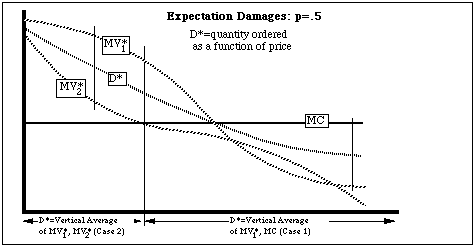Figure 2: Calculating the demand curve faced by a monopoly under a rule of expectation damages.

The conclusion of that analysis is that, for a given set of marginal value curves, there is some MCab such that for MC>MCab the reliance rule is superior to the expectation rule and for MC<MCab the expectation rule is superior to the reliance rule. It follows that neither rule is in general superior.

In working through situations such as that analyzed in Appendix I, it becomes clear why reliance is sometimes superior to expectation. For a given quantity ordered, expectation is superior because it results in the efficient quantity breached; units are breached only if their marginal value is less than the cost of completing them. But for a given quantity consumed, reliance is superior because it results in the efficient allocation of that quantity between consumers with MV1 and consumers with MV2--the allocation which makes the marginal value of the last unit consumed the same for all consumers.

Dé ja Vu: Damage Rules as Discriminatory Pricing

Some readers may suspect that they have seen some of this analysis somewhere before. They are probably correct. Both the analysis and the conclusions correspond to a special case of Pigou's third degree price discrimination. The seller of the previous section is in the same situation as a monopolist choosing prices in two separable markets, with the relation between the two prices constrained by the damage rule. The two markets consist of consumers in situation 1 with demand curve D1=MV1 and consumers in situation 2 with demand curve D2=MV2. Reliance damages correspond to a rule forbidding discrimination between the two markets; expectation damages correspond to a rule requiring the monopolist either to sell the same amount in both markets or to set the price in the market where the lower quantity is purchased equal to marginal cost.

Viewed as a special case of third order price discrimination, the results of the previous section are what one would expect. The standard efficiency argument against price discrimination is that charging the same price in all markets results in the efficient allocation of a given volume of output. That is the advantage of reliance damages. The standard efficiency argument for price discrimination is that under some circumstances it results in a larger volume of output, producing a benefit that more than balances the cost of inefficient allocation. Whether permitting price discrimination results, on net, in a better or worse outcome than forbidding it depends on the details of the demand and cost curves--as suggested by our examples.

Choice of Damages by the Monopolist: The Case Against Freedom of Contract

Figure 3 shows the situation of Figures 1 and 2 with one important difference: the monopolist is free to set his own damage rule. In keeping with the discussion of the previous section, the situation is graphed as a problem in discriminatory pricing. MR1,2 are the marginal revenue curves corresponding to all customers being in situations 1 and 2, respectively. The monopolist sells separate quantities Q1,2 in the two "markets," following the usual profit maximization rule: choose the quantity for which MC=MR. The total quantity Q+ is the average of the two, since each customer has an equal chance of being in either situation. Customers in situation 1 pay price P1, customers in situation 2 pay price P2.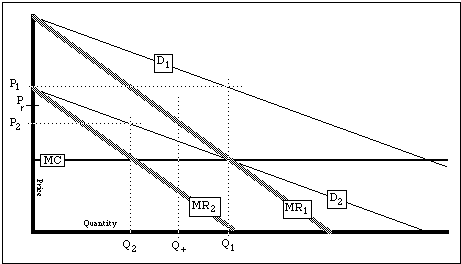Figure 3: Calculating the profit-maximizing damage rule and quantity, for a monopolist free to choose both, analyzed as a problem in discriminatory pricing.

How do we convert this into the language of damages for breach of contract? We have the prices on the separate margins of situations 1 and 2; we wish to deduce the corresponding price P for purchasing the good and damages D for breaching the contract. Viewed in terms of two markets, the cost of buying Q1 at a price P1 with a probability of .5 and Q2 at a price P2 with a probability of .5 is:

C(Q1,Q2)=[(P1)Q1+(P2)Q2]/2 (Equation 12)

Viewed in terms of contract and breach, the consumer orders Q1 units, paying P(Q1) for them. He then has a 50% chance of finding himself in situation 2, returning (Q1-Q2) units, and getting a refund of (P-D)--price minus damages.

C(Q1,Q2)=P(Q1)-(P-D)(Q1-Q2)/2

={ [2P-(P-D)]Q1+(P-D)Q2} /2 (Equation 13)

Equating the coefficients of Q1 and Q2 in Equation 12 with those in Equation 13, we get:

P2=P-D; P1=2P-(P-D)=2P-P2

Solving for P and D gives us:

P=(P1+P2)/2; D=(P1-P2)/2

So the monopolist sells at a price P=(P1+P2)/2 and charges damages for breach of (P1-P2)/2.

A rule of reliance damages corresponds, as pointed out before, to a rule requiring the monopolist to sell at the same price in both markets. The monopolist faces a demand curve which is the horizontal average of D1 and D2. From that demand curve he calculates a marginal revenue curve MR+. He maximizes his profit at the quantity at which MR+ intersects MC. The result is shown on Figure 4.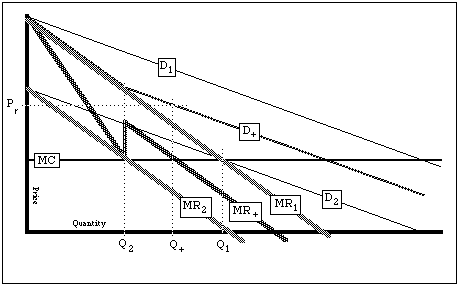Figure 4: Calculating the profit-maximizing quantity for a monopolist under a rule of reliance damages, analyzed as a problem in discriminatory pricing.

As can be seen from the figure, this is the same quantity, Q+, as in Figure 3. The amount produced is the same (for these demand and cost curves) under a legal rule of freedom of contract, in which the monopolist picks the damage rule that maximizes his profit, as under a rule in which damages are zero. The difference is that in the former case the monopolist, in effect, charges a high price (P1>Pr) to customers in situation 1, and a low price (P2<Pr) to those in situation 2. So freedom of contract leads to a less efficient allocation of the same output than does the reliance rule.

What about the expectation rule? The situation shown corresponds to MC<MCab, as the reader can easily check by comparing Figure 3 with the figures in Appendix I. That implies that expectation damages are superior to reliance damages, hence also superior to the outcome of freedom of contract.

So if the monopoly is free to specify damages in its sale contract, the result may not be the most efficient damage rule. This suggests a justification for courts refusing to accept damage rules agreed to by the parties, where one of the parties is a monopoly. That conclusion must be qualified by the observation that the court may lack the information or the incentives to produce a better rule. Even if the court can choose a better rule, it may be unable to enforce it; the court, after all, gets to rule on the contract only if the parties choose to go to court. An attempt to impose a damage rule other than that which would result from freedom of contract may merely result in costly--and successful--efforts by the seller to contract around it.

What if the monopolist is free to choose between the expectation and reliance rule, but not free to set any damage rule he likes? If MC>MCab, the monopolist operating under an expectation rule chooses to sell a quantity Qb* at a price MVb(Qb*). If Qb*<Qr, then we know from Figure 5 that MVa(Qb*)>MVb(Qb*); the monopolist operating under a reliance rule could sell the same quantity at a higher price yielding a higher profit, so he would prefer the reliance rule. If Qb*>Qr, on the other hand, then a monopolist operating under a reliance rule could also choose to sell Qb* at a price MVb(Qb*). The fact that he maximizes his profit by instead selling Qa* at a price MVa(Qa*) implies that the reliance rule is again preferred by the monopolist. Essentially the same argument can be used in the opposite direction for MC<MCab. It follows that for the situation where MV1,2 are parallel straight lines and R=0, or for any situation equivalent to it (remember that any R>0 situation can be transformed into an equivalent situation with R=0), freedom of contract leads to the efficient choice between expectation and reliance rules. How wide a range of other situations this result applies to I do not know.

The main purpose of this article is to analyze damage rules from the standpoint of economic efficiency. There is another way in which what we have just done can be viewed--one which carries us beyond the subject of this paper and into a more general discussion of monopoly pricing. One of the implications of this paper is that a monopolist may choose to sell goods in advance and charge damages for breach of contract even when reliance is zero and breach therefore costs him nothing.

Consider the situation from the standpoint of a monopolist choosing a damage rule. A reliance damage rule when reliance is zero is equivalent to letting the consumer decide whether to buy the good after he knows how much of it he wants. That rule does not maximize the monopolist's profit; he does better with advance contracts and a penalty for breach of contract . The combination of advance purchase and damages functions not to prevent inefficient reliance but as a device for price discrimination. It would be interesting to search for real-world examples of such a phenomenon.

Oligopoly, Bilateral Monopoly and Monopolistic Competition

So far, the analysis has been limited to the case of single-price monopoly--although the breach rule itself can be viewed as equivalent to charging different prices to two different groups of customers. The reason for concentrating on that case is not that it is the only form of imperfect competition where price is not equal to marginal cost but that it is the one most tractable to economic analysis. While I will not attempt any equally detailed examination of other forms of imperfect competition, it is worth saying a little about oligopoly, bilateral monopoly, monopolistic competition and discriminatory pricing.

In the case of both oligopoly and bilateral monopoly, equilibrium price and quantity are typically indeterminate, which makes it hard to discuss the relative efficiency of different rules. One can note that, just as with the simple monopoly we have discussed, the damage rule will affect the buyer's incentive to sign the contract as well as his incentive to breach it and that both effects should be taken into account in evaluating alternative rules.

A particularly simple case of bilateral monopoly occurs if one party is in a much better position to commit himself than the other. Suppose, for instance, that the seller is going to be in similar situations with other buyers many times, while the buyer will be in such a situation only once. The seller quotes a price and explains that he will stick to it whatever the buyer does. He can plausibly argue that to give in to the buyer's insistence on a lower price will weaken his bargaining position in future transactions with other buyers.

If the seller knows the value of the good to the buyer, he sets the price one cent below the full value and ends up with essentially all of the surplus. Suppose, however, that the seller has imperfect information about the buyer, in the form of a cumulative probability distribution [[rho]](P) showing the probability that the value of the good to the buyer is at least P.

The analysis of this situation is the same as the analysis of an ordinary single price monopoly. [[rho]](P), which shows the probability that a seller who insists on a price P will sell the good, corresponds to the demand curve D(P), which shows the number of units of the good the monopolist can sell at a price P. Marginal cost is the value of the good to the seller--the price at which he is indifferent between selling and not selling. Under these circumstances, the seller in bilateral monopoly is simply a monopolist whose (expected) quantity sold is between zero and one. The analysis of single price monopoly carries over intact and the conclusion is the same.

While single price monopoly is the simplest kind of imperfect competition to analyze, monopolistic competition is probably the most important in the real world. If we limit ourselves to firms that are unable to price discriminate, the analysis is very much the same. The one difference is that under monopolistic competition the density of firms is endogenous; the higher the profit an individual firm is able to get, the more firms crowd in, driving down the profit. So if two damage rules result in different profit levels for the same demand curves, they will, in equilibrium, result in different numbers of firms.

Shavell, Coase, and Perfect Discriminatory Pricing

Readers familiar with Shavell's 1980 paper on damages for breach of contract may at this point be wondering how its results can be consistent with those of this paper. Shavell, after considering breach under a variety of assumptions, concluded that "The expectation measure is Pareto superior to the reliance measure independent of the nature of the contractual situation." He further concluded that, in the situation where the same party decided about reliance and breach, "the expectation measure is ... a perfect substitute for a Pareto efficient complete contingent contract."A situation in which one party decides on breach and the level of reliance is fixed should be a trivial case of the same party deciding both. It seems to follow that, according to Shavell's analysis, the expectation rule not only dominates the reliance rule, it is the optimal rule for the cases we have been considering. Yet it seems clear from the analysis of this paper that it is not.

The reason for the discrepancy is that Shavell, in his paper, assumes away the central problem discussed in this one--the effect of the damage rule on the quantity initially purchased. He states (in a footnote) that "issues concerning contract formation (encompassing how parties meet and, if so, whether they reach agreement) are not studied here."He thus analyzes different damages rules while ignoring any effect they may have on whether the good is purchased in the first place. In effect, he assumes that the quantity initially sold is always efficient--or, at least, equally efficient under all of the alternative damage rules being considered. Under that assumption the problem I have been discussing--and, indeed, the standard efficiency problem of monopoly--vanishes.

One way of defending Shavell's assumption is to assume that the Coase Theorem applies to the formation of a contract but not to its breach. If the Coase Theorem applies to making a contract, then we can ignore any effect of damage rules on what contracts get made; whatever the damage rule, all contracts which produce net gains will be signed and only such contracts. If the Coase Theorem also applied to breaching a contract, we could ignore damage rules there as well; whatever the rule, only efficient breach would occur. But if the Coase Theorem does not apply to breach, then damage rules affect the decision to breach, and the expectation rule leads to the efficient outcome.

While this is a possible set of assumptions, it does not seem a particularly plausible one. There is no particular reason why, if buyer and seller can bargain to an efficient outcome in drawing up the original contract, they cannot also bargain to an efficient outcome when one party wishes to breach. It seems more reasonable to assume that the Coase Theorem applies to both parts of the transaction or neither. In the former case, the outcome is always efficient and the damage rule is irrelevant. In the latter case, which we have been assuming in this article, both formation and breach are conducted without individual bargaining. The result is the standard textbook model of monopoly. The seller sets one price at which all customers may buy. The buyer decides whether to breach according to the costs implied by the applicable legal rule.

Shavell does not discuss whether the market he is discussing is competitive or monopolistic or why expectation and reliance rules lead to different results. He is concerned with the situation only after the contract has been formed--at which point those questions are no longer relevant. Another way of justifying his approach might be to assume that the market he discusses is a competitive one and to argue that under perfect competition the market price already reflects any cost that the buyer may impose on the seller by buying with some probability of breaching. With the quantity on one margin optimized by the price one can then use an expectation damages rule to optimize the quantity on the other margin.

In the next section, we will see to what degree that argument holds. Before doing so, it is worth nothing that there is at least one form of imperfect competition for which the assumption necessary to Shavell's analysis is correct and to which his conclusions therefore apply.

In the usual monopoly context of one seller and many buyers, Shavell's assumption would be true if the seller engaged in perfect discriminatory pricing--charging each buyer for each unit the highest price he is willing to pay. Under perfect discriminatory pricing the quantity produced is efficient, since the seller can and will cut price on marginal units all the way down to marginal cost without losing revenue on his higher priced inframarginal units. Under such circumstances, freedom of contract would lead to expectation damages and would be efficient. The seller would choose the damage rule that set the marginal cost to the consumer in situation 2 at its efficient level (MC), and set the purchase price (differently for each unit sold to each consumer) at the level that collected all of the resulting surplus.

IV: Perfect Competition with Uncertain Cost

So far, we have only considered imperfect competition. Reliance and expectation rules lead to different conclusions because price is not equal to marginal cost; price is not equal to marginal cost because the market is not competitive.

A difference between price and marginal cost might also arise in a perfectly competitive market with uncertainty, where the seller discovered his production cost only after contracting to produce the good. The contract price would equal average cost ex ante, so average profits would be zero. By the time the buyer decided whether to breach, however, both parties would know the actual cost of completing the good. Since it would generally not equal the contract price, a reliance rule would produce a different result than an expectation rule.

In choosing between the two rules, one crucial question is who knows what when. In the case of symmetric information, where, at the time the contract is signed, both buyer and seller have the same information about the probability of cost and of breach, it is simple to show that expectation damages not only are superior to reliance damages but result in an efficient outcome. In other situations, the advantage of an expectation rule (efficient incentive for the buyer to breach) must be balanced against the advantage of a reliance rule (efficient incentive for the buyer to include the cost of ordering and then breaching when he decides how much to order). But with symmetric information the seller has the same information about the potential costs of sale followed by breach as the buyer, so those costs are fully reflected in the purchase price, leading to an efficient quantity ordered even under an expectation rule.

If all sellers know (and buyers do not know) the cost of producing units for a particular buyer then in a competitive market the varying costs will be reflected in the prices charged to different buyers; price equals cost ex post as well as ex ante and the distinction between expectation and reliance damages vanishes.

A reliance rule gives buyers the efficient incentive with regard to ordering the units they are going to breach. Buying and then breaching imposes a cost of R (the amount of reliance) on the seller, which is what the buyer must pay as damages. Under either reliance or expectation, the buyer still has an inefficient incentive with regard to ordering units he is going to accept. All buyers are charged a price reflecting the average cost of producing for both high cost and low cost customers, so high cost customers buy more than the efficient quantity and low cost customers less. Once a unit is ordered, the reliance rule gives high cost customers an inefficiently high and low cost customers an inefficiently low incentive to breach; the expectation rule gives the correct incentive. The analysis is worked out formally in Appendix II.

The conclusion, here as in the discussion of imperfect competition, is that there are advantages to both rules. The reliance rule gives the buyer the correct incentive to avoid breaching a contract by not signing it--or, more generally, to take into account the cost of buying and then breaching in deciding how much to buy. The expectation rule gives the buyer the correct incentive to avoid breach by fulfilling the contract once it is entered into.

Under both perfect and imperfect competition we can expect one rule to be unambiguously superior to the other if we have some reason to expect that one decision or the other--the decision to buy or the decision to breach--will be made efficiently under both rules. Under imperfect competition, the decision to buy is made efficiently with perfect discriminatory pricing or perfect Coase theorem bargaining; in those situations expectation damages are unambiguously superior. Under perfect competition, the same is true for symmetric information. Similarly, under perfect competition, reliance damages are superior for all-or-nothing breach--the situation where, if the event which might trigger breach occurs, the value of the good drops to zero and the purchaser breaches the entire contract under either rule.

V: Conclusion

In this paper, I have tried to establish two things. The first is that, in order to evaluate different damage rules correctly, one must consider their effect on the decision to buy as well as on the decision to breach, since failing to sign a contract is one way of avoiding damages for breaching it. The second is that, once one includes that effect, the case for the general superiority of expectation damages disappears.

In establishing these results, I have looked at two large classes of problems--imperfect competition and perfect competition with uncertain costs. Within each class, I have tried to establish general principles by looking at a variety of possible situations.

In the case of imperfect competition, one conclusion is that the analysis of damage rules can be viewed as an application of the theory of third order discriminatory pricing. A rule of reliance damages is like a rule prohibiting discriminatory pricing; the arguments for the efficiency of reliance damages, and the limitations to those arguments, are the same as for the efficiency of a prohibition on price discrimination.

In discussing perfect competition I have limited myself to situations in which production cost is uncertain, since with perfect competition and full information there are no profits to be lost and expectation and reliance rules therefore yield the same damages.The results of the analysis then depend on whether buyer and seller have the same information.

In the case of symmetric information, the market price includes all the information about costs of breach available at the time the contract is signed. There is no advantage to reliance damages, since when the buyer is deciding how much to order he knows nothing relevant to breach that the seller does not. The results of Shavell (1980) can then be expected to hold, just as they can be expected to hold for perfect price discrimination and for the same reason.

If the buyer knows more about the cost of production than the seller, however, inefficient ordering is a potential problem and a reliance rule serves a useful function in limiting it. The benefit of the reliance rule in generating an efficient quantity ordered given the (inefficient) level of breach anticipated must be balanced against the benefit of the expectation rule in generating an efficient level of breach given the (inefficient) quantity ordered.

In establishing these results, I have deliberately avoided many of the complications associated with the issue of damages for breach, both in the real world and in the existing literature. My purpose was to show that even in simple cases the conventional analysis omitted an essential point and, as a result, reached a conclusion which was, in many situations, incorrect.

What do these results imply about how an efficient legal system would deal with breach of contract? The clearest implication is that the presumption in favor of expectation damages should be limited to cases in which the contract is formed in a competitive market with symmetric information between buyer and seller. Beyond that, the most one can say is that an expectation rule is a solution to the problem of inefficient breach and a reliance rule a solution to the problem of inefficient purchase. Which is more appropriate for a particular contract will depend on which problem is more likely to be serious--a matter which courts may or may not be competent to judge.

Appendix I

We consider the choice between reliance and expectation rules in the case of a single price monopoly. The consumer faces two states of the world, 1 and 2, with probabilities (1-p) and p. In state i, his marginal value for the good is MVi. Reliance equals zero. Marginal cost is constant at MC, fixed cost is zero. We assume:

MV1(Q)=A-BQ; MV2(Q)=A-E-BQ; A,B,E>0

We define:

Qr= (1-p)E/B; Qe= (A-MC-E)/B

MVa= A-BQ/(1-p)

MVb= A-BQ-pE

MVc= (1-p)(A-BQ)+pMC

As shown earlier, Da(P), the inverse function of MVa(Q) (quantity as a function of price rather than marginal value as a function of quantity) is the demand curve under a reliance rule for Q<Qr, Db(P) is the demand curve under a reliance rule for Q>Qr and is also the demand curve under an expectation rule for Q<Qe, and Dc(P) is the demand curve under an expectation rule for Q>Qe. As earlier, Q is the quantity consumed under a reliance rule but the quantity ordered under an expectation rule.

Profit will be maximized where MR=MC. We therefore define:

Qa*: MRa(Qa*)=A-2BQa*/(1-p)=MC; Qa*=(1-p)(A-MC)/2B

Qb*: MRb(Qa*)=(A-pE)-2BQb*=MC; Qb*=(A-MC-pE)/2B

Qc*: MRc(Qc*)=[(1-p)A+pMC]-2(1-p)BQc*=MC; Qc*=(A-MC)/2B

We wish to discover the value of MC such that, under a reliance rule, the producer will be indifferent between producing to the left of Qr on MVa or to the right of Qr on MVb; we will call it MCab. Defining TRa,b,c as the integral of MRa,b,c, we have, for MC=MCab:

B(Qr-Qa*)2/(1-p)=TRa(Qa*)-TRa(Qr)=TRb(Qb*)-TRb(Qr)=B(Qb*-Qr)2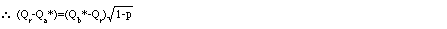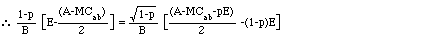With a little algebraic manipulation, this yields:We define Qr*(MC) as the profit-maximizing quantity for a producer under a reliance rule. If MC>MCab then Qr*=Qa*; if MC<MCab then Qr*=Qb*.

Next we wish to discover the value of MC such that, under an expectation rule, the producer will be indifferent between producing to the left of Qe on MVb or to the right of Qe on MVc; we will call it MCbc.We have, for MC=MCbc:

B(Qe-Qb*)2=TRb(Qb*)-TRb(Qe)=TRc(Qc*)-TRc(Qe)=B(1-p)(Qc*-Qe)2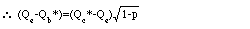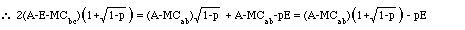Which simplifies to: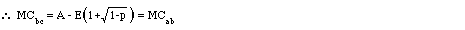For A-pE>MC>MCab we therefore have Qr*=Qa*, Qe*=Qb*; for MC<MCab we have Qr*=Qb*, Qe*=Qc*. For MC>A-pE Qr*=Qa*, Qe*=0.

We next wish to find out which rule is superior--which leads to a higher total of consumer surplus plus profit. We have, for the case MC>MCab: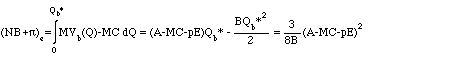So reliance is superior <=>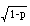(A-MC)>A-MC-pE

Substituting in our value for MCab, we find that(A-MC)=A-MC-pE for MC=MCab, so(A-MC)>A-MC-pE for MC>MCab. It follows that the reliance rule is superior for MC>MCab.28

For MC<MCab: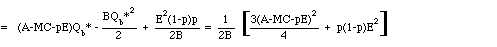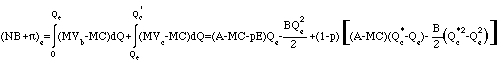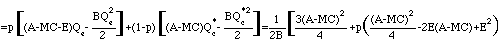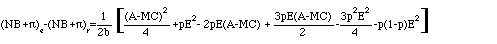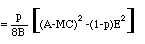If we set MC=MCab, this last term is positive, so expectation is superior to reliance. The same is true a fortiori for MC<MCab. It follows that reliance dominates for MC>MCab and expectation dominates for MC<= MCab.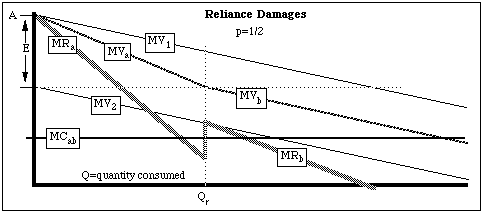Figure 5: The analysis of a monopoly selling under a rule of reliance damages, as modeled in Appendix I. MVa and MRa are only shown for values of Q to the left of Qr; MVb and MRb are only shown for values of Q to the right of Qr. The demand curve and the resulting marginal revenue curve faced by the monopolist are identical to the parts of MVa,b and MRa,b shown.Figure 6: The analysis of a monopoly selling under a rule of expectation damages, as modeled in Appendix I. MVb and MRb are only shown for values of Q to the left of Qe; MVc and MRc are only shown for values of Q to the right of Qe. The demand curve and the resulting marginal revenue curve faced by the monopolist are identical to the parts of MVb,c and MRb,c shown.

Appendix II

We consider the choice between reliance and expectation damage rules in a perfectly competitive market with asymmetric information. We assume, as before, marginal values MV1,2, reliance R, probability of the event leading to breach p. Marginal cost is MCa with probability pa, MCb with probability pb, pa+pb=1, MCb<MCa. The consumer knows marginal cost before the goods are purchased; the producer does not. Since the industry is perfectly competitive, average profit is zero.

The analysis of consumer behavior in part II applies to this situation as well; the initial problem may be converted into an equivalent problem with transformed marginal value curves and zero reliance.

We must now introduce explicitly a constraint which was earlier ignored. Under expectation damages a breaching consumer must make the seller as well off as if there had been no breach. If the seller's expected profits from completing the contract are negative, a literal interpretation of the expectation rule would imply negative damages; the buyer who breaches part or all of the contract receives not only a refund but an additional payment as a reward for breaching. While that is a literal interpretation of the definition of expectation damages, it is not one that a court would follow; you cannot recover on the grounds that your breach benefitted the other party.

We therefore impose the additional constraint that damage payments must be non-negative. In the case of imperfect competition this constraint is never binding, since the monopolist never chooses a price at which his profits are negative. But in the case of asymmetric information, the seller may find that, ex post, he is receiving negative profits and would be better off if the buyer breached. So expectation damages equal P-(MC-R) only for P>= MC-R; otherwise they are zero.

When we transform the problem from MV1,2, R to MV*1,2,0, we do not change P or MC, so the constraint still contains the old value of reliance (R). The usual definition of expectation damages applies only for P>= MC-R. In the analysis below, I assume R large enough so that we can ignore the problem of negative damages.

Reliance

Figure 7 shows the relevant curves. The seller charges a price Pr. The consumer purchases a quantity Q1. If the event occurs which lowers his marginal value curve to MV2, he breaches a quantity Q1-Q2 and accepts delivery on a quantity Q2. Under the reliance rule, the actual value of MC is of no importance to the buyer since it does not affect the damages he must pay for breaching, so neither Q1 nor Q2 depends on it.

From the zero profit condition, we have:

[[pi]] =[(1-p)Q1+pQ2][Pr-paMCa-pbMCb]=0

\ Pr=paMCa-pbMCb

Since there is no penalty for breach (we are dealing with the transformed problem, so reliance is zero), the consumer can pick Q1 and Q2 separately to maximize his surplus from MV1 and MV2. He does so by choosing the values for which MV1 (Q1)=Pr=MV2(Q2). The result is shown on the figure.

Expectation

Under expectation damages, the situation is more complicated. Damages are equal to P-MC. Since the buyer knows the value of MC in advance, he will buy different quantities in case a (high marginal cost) than in case b. I accordingly define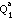,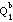,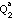andas the corresponding quantities. We have:

Net Benefit=(1-p){ pa[TV1()-Pe]+pb[TV1()-Pe]} (value minus price in situation 1)

+ p{ pa[TV2()-Pe]+pb[TV2()-Pe]} (value minus price in situation 2)

- { pa[-][Pe-MCa] + pb[-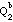][Pe-MCb]} (damage payment)

=(1-p){ pa[TV1()-(Pe +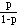(Pe-MCa))]+pb[TV1()-(Pe +(Pe-MCa))]

+ p{ pa[TV2()-MCa]+pb[TV2()-MCb]}

Maximizing net benefit with respect to,,andgives us:

MV1()=(Pe +(Pe-MCa))

\ MVa()= [MV1()+MCa]/[1+] = Pe

MV1()=(Pe +(Pe-MCb))

\ MVb()= [MV1()+MCb]/[1+] = Pe

MV2()=MCa

MV2()=MCb

The zero profit condition is:

[[pi]] =pa[Pe-MCa] + pb[Pe-MCb] = 0

\ Pe= [paMCa+pbMCb]/[pa+pb] > Pr

The final inequality follows from the fact that>. The customer knows that his damage payment in case of breach is lower when marginal cost is higher, so he orders more units. Since the seller knows that high cost customers order more than low cost customers, he must set price above the average of MCa and MCb in order to cover cost. Under reliance, high cost and low cost customers order the same amount, so price is simply the average of MCa and MCb.

The result is shown on Figure 7. The lightly hatched area A is the increased surplus due to using a reliance rule, the diagonally hatched area B is the increased surplus due to an expectation rule. If A>B the reliance rule is superior, if A<B the expectation rule is superior.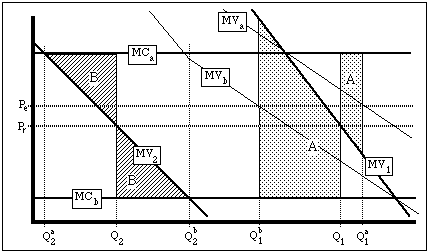Figure 7: Consumer surplus with reliance (A) and expectation (B) rules under the model of Appendix II.

Looking at the figure, it is clear where the advantages of each rule come from. The expectation rule produces the optimal level of breach, giving a gain in surplus of B, but it produces an inefficient pattern of ordering (more ordered when cost is higher) giving a loss in surplus of A.

In drawing the figure, I assumed for simplicity>and>, avoiding corner solutions. I also assumed>= Q1. If<Q1 then the area above MV1 betweenand Q1 represents negative surplus. It is straightforward to show that A is still positive in this situation, so the reliance rule still results in a more efficient quantity ordered.

All-or-Nothing Breach

One interesting special case occurs when MV2= 0. If the event occurs, all units of the good are useless to the buyer, so under either damage rule he refuses to take delivery of any of them. Area B then disappears, making the reliance rule unambiguously superior. The advantage of the expectation rule, as pointed out before, is that it leads to an efficient level of breach. In this situation both rules lead to the efficient level of breach, so the expectation rule has no advantage. This is just the reverse of what happens under perfect discriminatory pricing or symmetric knowledge (under perfect competition); in those situations both rules lead to an efficient quantity ordered, so the expectation rule is unambiguously superior.

Back to the list of articles.